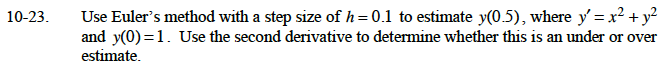### Home > CALC > Chapter 10 > Lesson 10.1.3 > Problem10-23

10-23.Since h = 0.1, this is equivalent to dx = 0.1.
Therefore dy = 0.1(x2 + y2).

Starting at (0, 1), dy = 0.1, so the next point is (0.1, 1.1).

Starting at (0.1, 1.1), dy = 0.122, so the next point is (0.2, 1.222).

Now repeat this process three more times.